Function Repository Resource:

HexagonalSpiralPoints

Get the coordinates of the points on a hexagonal spiral

Contributed by: George Beck
 ResourceFunction["HexagonalSpiralPoints"][n] gives the list of coordinate pairs on a hexagonal spiral with n sides starting with the origin.

Details and Options

The list starts at the origin and roughly goes NE, NW, W, SW, SE, E and repeats.

Examples

Basic Examples

Here are the points on the first four legs of the spiral:

 In:=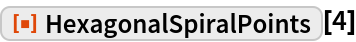Out=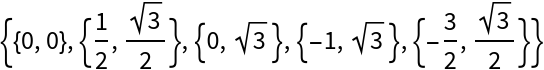This shows the sequence of points in order for six sides:

 In:=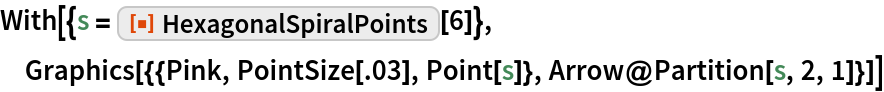Out=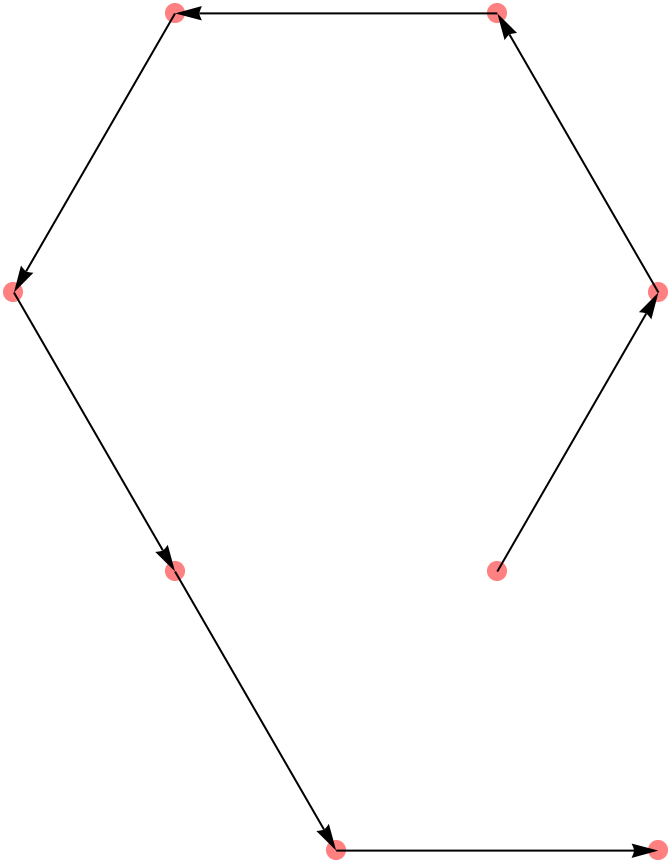Forty black sides with 20 red sides overlaid:

 In:=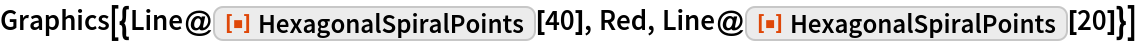Out=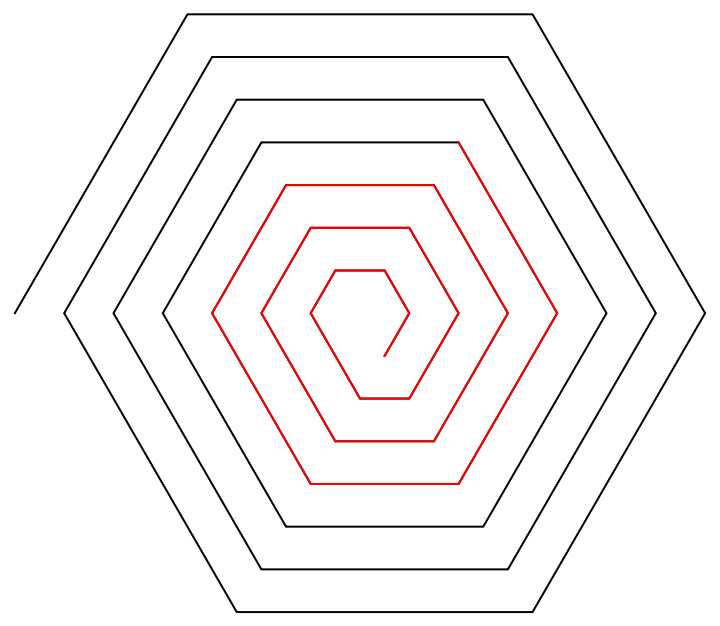The number of points in the first n sides:

 In:=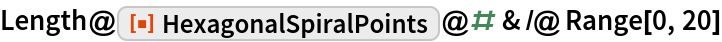Out=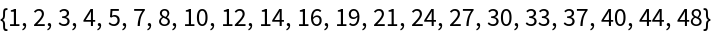In:=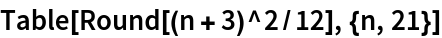Out=Neat Examples

This finds the coordinate pairs that are a prime distance, counting along the square spiral:

 In:=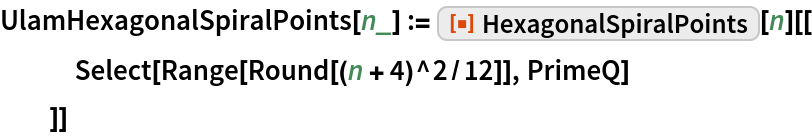The larger points correspond to the primes 2, 3, 5, 7, 11, 13:

 In:=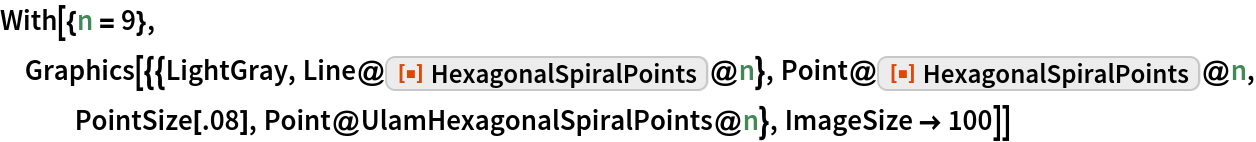Out=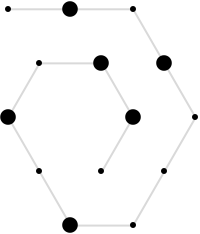About 12% of the numbers up to 10443 are prime:

 In:=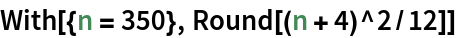Out=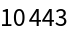In:=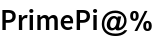Out=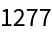Here is a plot of the first 1277 primes:

 In:=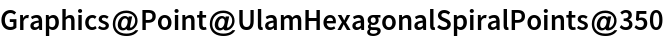Out=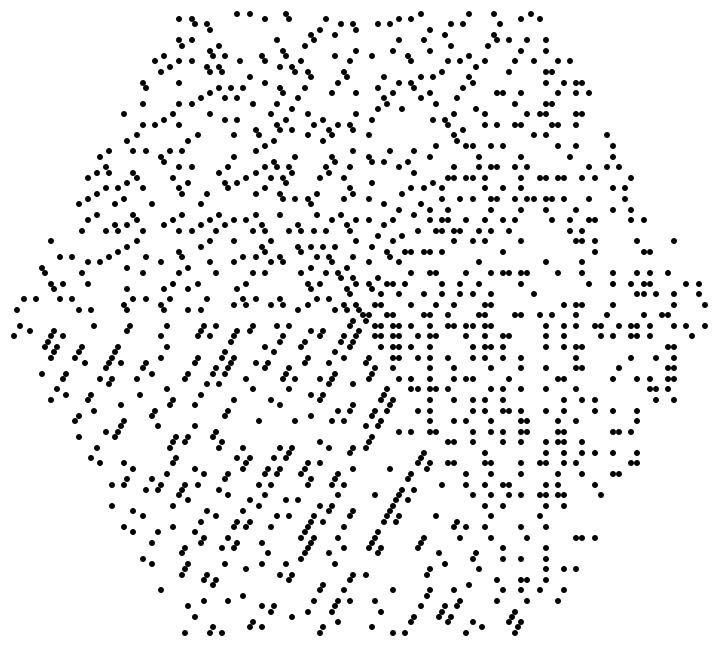About 11% of the numbers to 10443 are lucky:

 In:=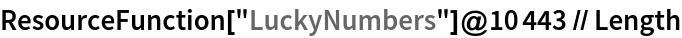Out=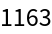This finds the coordinate pairs that are at lucky number distances along the hexagonal spiral:

 In:=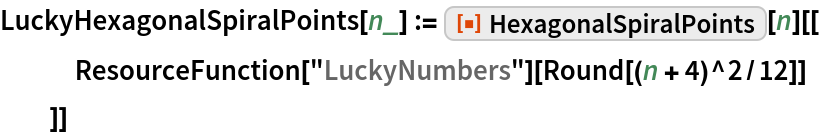Here are the first 1248 lucky numbers plotted along the hexagonal spiral:

 In:=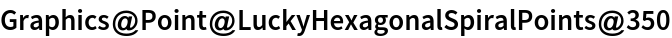Out=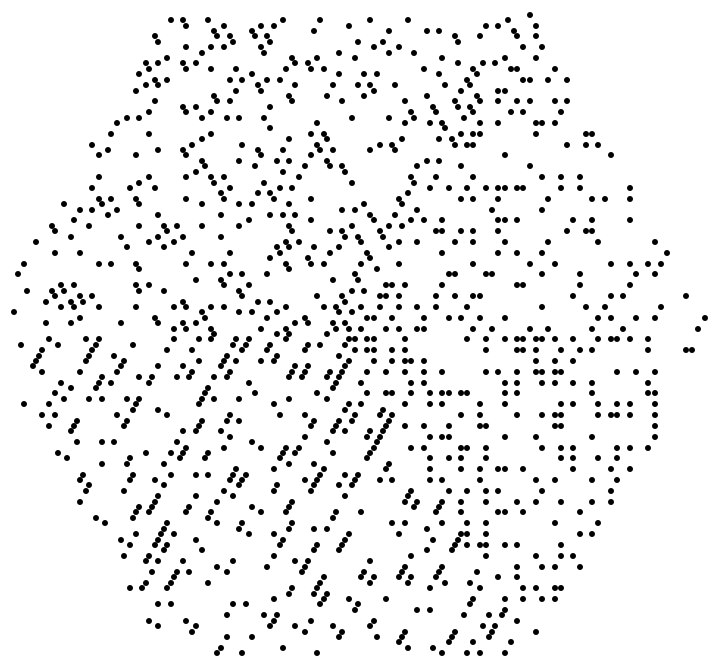Requirements

Wolfram Language 11.3 (March 2018) or above